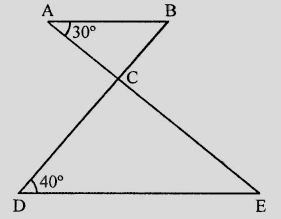"
">

# In the figure, $AB \parallel DE$, find $\angle ACD$."

Given:

In the given figure, $AB \parallel DE$.

To do:

We have to find $\angle ACD$.

Solution:

$AE$ and $BD$ intersect each other at $C$

$\angle BAC = 30^o$ and $\angle CDE = 40^o$

$AB \parallel DE$

This implies,

$\angle ABC = \angle CDE$                     (Alternate angles)

$\angle ABC = 40^o$

In $\triangle ABC$, $BC$ is produced

$\angle ACD = \angle CAB + \angle ABC$

$= 30^o + 40^o$

$= 70^o$

The value of $\angle ACD$ is $70^o$.

Updated on: 10-Oct-2022

33 Views